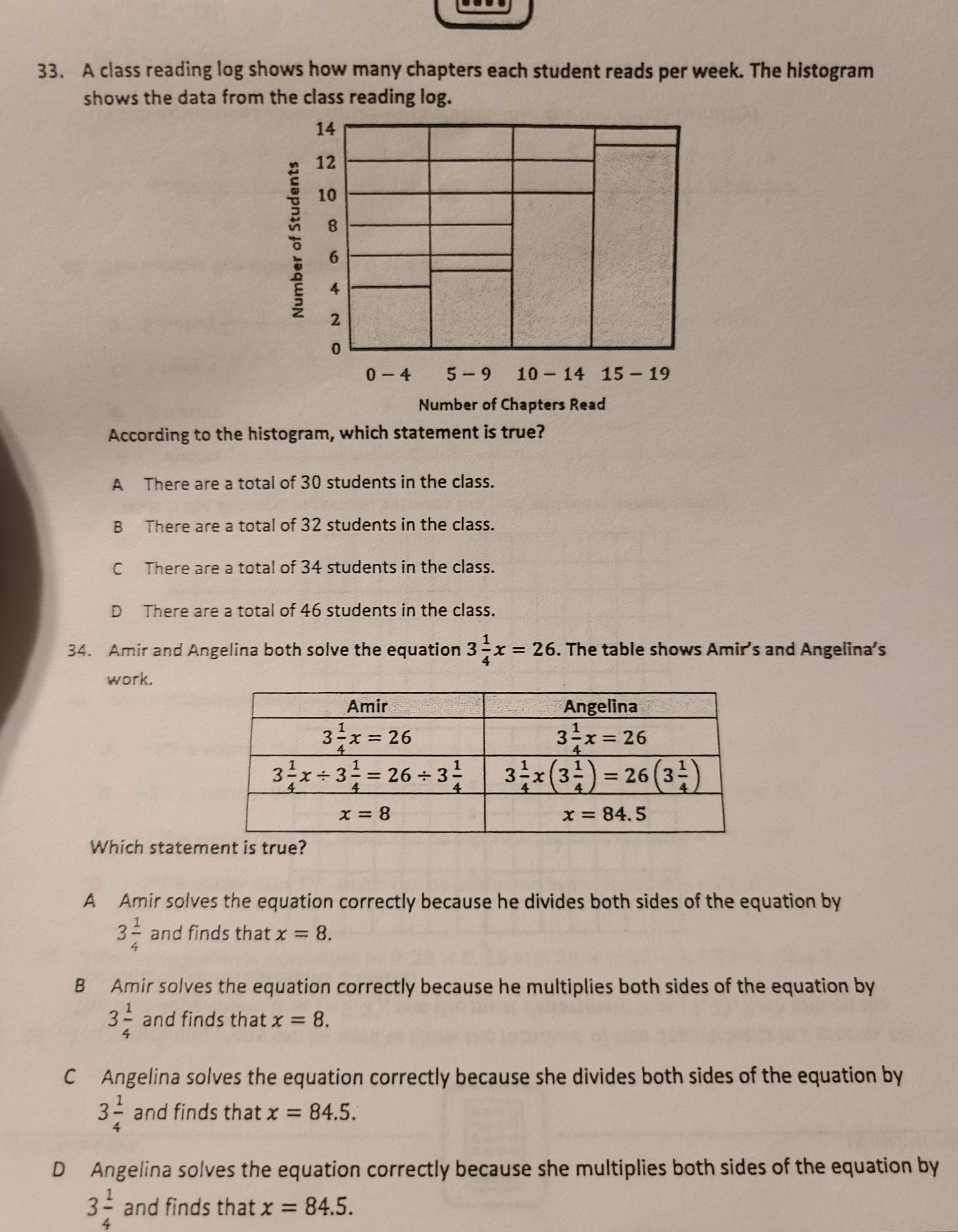### ¿Todavía tienes preguntas de matemáticas?

Pregunte a nuestros tutores expertos
Algebra
Pregunta33. A class reading log shows how many chapters each student reads per week. The histogram shows the data from the class reading log. According to the histogram, which statement is true?

A There are a total of $$30$$ students in the class.

B There are a total of $$32$$ students in the class.

C There are a total of $$34$$ students in the class.

D There are a total of $$46$$ students in the class.

34. Amir and Angelina both solve the equation $$3 \frac { 1 } { 4 } x = 26 .$$ The table shows Amir's and Angelina's work. Which statement is true?

A Amir solves the equation correctly because he divides both sides of the equation by $$3 \frac { 1 } { 4 }$$ and finds that $$x = 8$$ .

B Amir solves the equation correctly because he multiplies both sides of the equation by $$3 \frac { 1 } { 4 }$$ and finds that $$x = 8$$ .

C Angelina solves the equation correctly because she divides both sides of the equation by $$3 \frac { 1 } { 4 }$$ and finds that $$x = 84.5$$ .

D Angelina solves the equation correctly because she multiplies both sides of the equation by $$3 \frac { 1 } { 4 }$$ and finds that $$x = 84.5$$ .